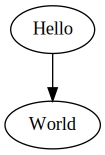Coding Interview Tips

First things first, ask questions before you jump into coding.

Draw Diagrams

If required, and only if required, draw diagrams.Ofast

#pragma GCC optimize("Ofast")
#pragma GCC optimization("fast-math")
#pragma GCC optimization("unroll-loops")
#pragma GCC optimization("max-inline-insns-recursive-auto")
#pragma GCC target("avx,avx2,fma")

static const int _=[](){std::ios::sync_with_stdio(false);cin.tie(nullptr);cout.tie(nullptr);return 0;}();

Built-in Functions

__builtin_popcount
__builtin_parity
__builtin_clz
__builtin_ctz

Overflow

See the reverse integer problem for some basic overflow avoidance logic. And the sqrt(x) problem for more advanced overflow when you need to take into account the division and the denominator can be zero so that has to be treated separately.

Underflow

a.size() - b.size() can underflow because they're both size_t, so you must do (int)(a.size() - b.size()).

Freeing up Nodes

It's often forgotted, but when you remove a node from a linked list, you must free up its memory. Use delete.

Two's Complement

To calculate two's complement of a negative number in C++, do (uint)num -- it's as simple as that.